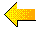STAT LABS: Frequently Asked Software Questions

HOW DO I...

We have had good results using S-Plus and R in our courses to analyze Stat Labs data. Currently we are using R because it is free, and versions are available for the unix, Mac, and PC environments. The syntax of the two languages are nearly identical.

R can be downloaded from The Comprehensive R Archive Network, where you can also find a User's Guide. We also provide here a short list of comonly used commands, and their syntax.

Splus is available from Insightful, and a free student version is available from this link. Instructions for the student version are available here.

We also recommend Introductory Statistics with R by P. Dalgaard and
An Introduction to S and S-Plus by P. Spector.

FAQ:  Here you will find answers to our students' frequently asked questions on how to use R and Splus. The responses provided do not comprise a comprehensive user's guide to the R or S-plus language. Refer to the user's guides listed on this page for a more thorough introduction to these languages.

The following examples all use the birth weight data, called babies. It contains variables bwt (birth weight in ounces), smoking (0 for nonsmoker, 1=yes now, 2=until pregnant, 3=once did not know, 9=unkown), wt (mother's prepregnancy weight), educ (categories 0-7, 9=unknown).

• Getting data from the web into R
• Replacing values
The 999 values in bwt denote missing values. To replace 999 with NA.
replace(bwt,bwt==999,NA)
The 9 values in smoke denote missing values. To replace 9 with NA and recode 2 and 3 as 0, for nonsmoker:
ismoke<- replace(smoke,smoke==2 |smoke==3,0)
ismoke[ismoke==9]<-NA

To convert the values of gestation into weeks, and to also collapse these values into 5 catgories,
gestwks<-floor(gestation/7)
gestcut<-cut(gestwks,5)
• Creating matrices and vectors
To create a matrix of 0s and 1s, where there is one column for each education level, and the 1s in that column indicate the mother has that education level.
outer(educ,unique(educ),"==")
To create a 3 by 4 matrix of all 0s.
matrix(0,nrow=3,ncol=4)
To create an array (0, 0.1, ..., 0.9, 1).
seq(from=0,to=1,by=0.1)
To create an array (1,1,1,12,34,34).
c(1,1,1,12,34,34) or
rep(c(1,12,34),c(3,1,2))
• Data frames, matrices, and lists
• Computing statistics on subgroups
To compute the average birthweight for smokers and nonsmokers.
tapply(bwt,ismoke,mean)
To compute the average birthweight for mothers who are above average in weight.
tapply(bwt,(wt > mean(wt)),mean)
• Selecting a subset
To select babies whose mother smoked.
smokerbabes<-babies[is.na(ismoke) | ismoke==1]

• To count babies according to whether they are premature or low birthweight:
table(cut(gestation,4),bwt<90)
To find the mean of height and weight for mothers according to education and smoking status.
apply(cbind(ht,wt),2,function(x)tapply(x,list(educ,ismoke),mean)
To regress bwt on mother's weight for mothers separatley for smokers and nonsmokers
reg<-tapply(seq(length(bwt),ismoke,function(x)lm(bwt[x]~wt[x]))
lapply(reg,coeff)
• Multiple plots with the same axes
To make two histograms, one for smokers and on for nonsmokers, where they both have the same axes.
par(mfrow=c(2,1))
tapply(bwt,ismoke,hist,xlim=c(min(bwt),max(bwt)),ylim=c(0,50))

or
par(mfrow=c(2,1))
hist(bwt[ismoke==1],xlim=c(min(bwt,max(bwt))
par(xaxs="d",yaxs="d")
hist(bwt[ismoke==0])
• Quantile plots
To make a quantile-quantile plot of bwt for smokers versus nonsmokers
qqplot(bwt[ismoke==0],bwt[ismoke==1])
To make a gamma(5,1) quantile plot of bwt
ps<-ppoints(length(bwt))
plot(quantile(bwt,ps),qgamma(ps,5,1))
• Multiple boxplots on one plot
To put box and whisker plots of bwt, one for each education level, on the same plot
boxplot(bwt~educ)
• Putting curves on plots
To make a plot of bwt by wt, and place on it a curve that is computed from a function g:
plot(wt,bwt)
pts<-seq(from=par("usr"),to=par("usr"),len=100)
lines(pts,g(pts),xpd=T) <-seq(from=par("usr"),to=par("usr"),len=100)
• Sliding bin plots
• Contour plots
• Nonparametric bootstrap
Here we will use the data from the video game survey. The sample size is 91, and the population size is 314. We first create a bootstrap population of time. Then we take a simple random sample of 91 from the bootstrap population 100 times.
dups<-round(table(time)*314/91)
bootpop<-rep(cbind(unique(time),dups)
m<-rep(0,100)
for( in 1:100){s<-sample(bootpop,size=91,replace=F);m[i]<-mean(s)}
• Parametric bootstrap
Here we take 100 stratified randoms from a normal distribution, where there are two strata. One strata has a mean of 10 and sd of 1, and the second has a mean of 0 and sd 1. Each sample will consist of 10 observations from the first stratum and 15 from the second.
means<-rep(c(10,0),c(10,15))
apply(matrix(0,nrow=100,ncol=1),1,function(x)rnorm(n=25,mean=means))About Stat Labs How to Use Stat Labs Stat Labs Data Software To Order

last updated on March 21, 2000.